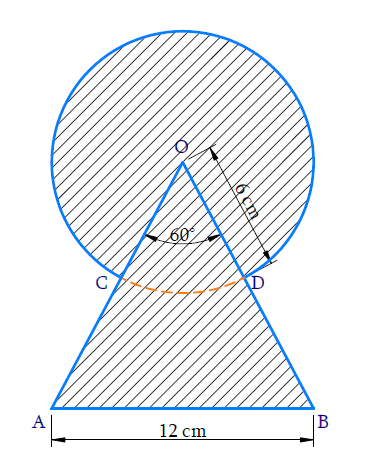# Ex.12.3 Q4 Areas Related to Circles Solution - NCERT Maths Class 10

Go back to  'Ex.12.3'

## Question

Find the area of the shaded region in the given figure, where a circular arc of radius $$\text{6 cm}$$ has been drawn with vertex $$O$$ of an equilateral triangle $$OAB$$ of side $$\text{12 cm}$$ as centre.Video Solution
Areas Related To Circles
Ex 12.3 | Question 4

## Text Solution

What is known?

A circular arc of radius $$\text{= 6 cm}$$ is drawn with vertex $$O$$ of an equilateral $$\Delta {OAB}$$ of side $$\text{12 cm}$$ as centre.

What is unknown?

Reasoning:

From the figure it is clear that the shaded region has an overlap region of area of a sector of angle $${60^ \circ }$$ (since each angle of an equilateral $$\Delta$$ is of measure $${60^ \circ }$$ area of a circle with radius $$\text{6 cm}$$ and area of triangle $$OAB$$ with side $$\text{12 cm.}$$

$$\therefore\;$$Area of shaded region $$=$$ Area of circle with radius $$\text{6 cm}\; +$$ Area of $$\Delta {OAB}$$ - Area of sector of angle $${60^ \circ }$$

\begin{align} = \pi {(6)^2} + \frac{{\sqrt {3{{(12)}^2}} }}{4} - \frac{{{{60}^\circ}}}{{{{360}^\circ}}} \times \pi {(6)^2}\end{align}

Using formula, Area of an equilateral \begin{align} \Delta = \frac{{\sqrt 3 }}{4}{({\mathop{\text sid}\nolimits} e)^2}\end{align}

Area of the sector of angle \begin{align} \theta = \frac{\theta }{{{{360}^\circ }}} \times \pi {r^2} \end{align}

Where $$r$$ is the radius of the circle

Steps:

Radius of circle $$(r) =\text{ 6 cm}$$

Side of equilateral $$\Delta {OAB(s)} = 12\,{\text{cm}}$$

We know each interior angle of equilateral $$\Delta = {60^\circ }$$

Since they overlap, part of area of a sector $$OCD$$ is in area of the circle and triangle.

$$\therefore \;$$ Area of shaded region = Area of circle $$+$$ Area of $$\Delta {OAB}\,-$$Area of sector $$OCD$$ of angle $$60^\circ$$

\begin{align} &= \pi {\left( 6 \right)^2} + \frac{{\sqrt 3 }}{4}{\left( {12} \right)^2} - \frac{{60}}{{360}} \times \pi {\left( 6 \right)^2}\\ &= 36\pi + 36\sqrt 3 - \frac{{36}}{6}\pi \\ &= 36\left( {\pi + \sqrt 3 - \frac{\pi }{6}} \right)\\ &= 36\left( {\sqrt 3 + \frac{{5\pi }}{6}} \right)\\ &= 36\sqrt 3 + 30\pi \\ &= 36\sqrt 3 + 30 \times \frac{{22}}{7}\\ &= 36\sqrt 3 + \frac{{660}}{7}\,{\text{c}}{{\text{m}}^2}\end{align}

Learn from the best math teachers and top your exams

• Live one on one classroom and doubt clearing
• Practice worksheets in and after class for conceptual clarity
• Personalized curriculum to keep up with school